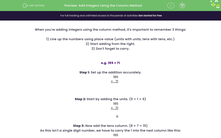# Add Integers Using the Column Method

In this worksheet, students will practise using the column method in addition sums involving up to 3 digits and 3 numbers.Key stage:  KS 4

Year:  GCSE

GCSE Subjects:   Maths

GCSE Boards:   Pearson Edexcel, OCR, Eduqas, AQA,

Curriculum topic:   Number, Number Operations and Integers

Curriculum subtopic:   Structure and Calculation Calculations with Integers

Difficulty level:#### Worksheet Overview

When you're adding integers using the column method, it's important to remember 3 things:

1) Line up the numbers using place value (units with units, tens with tens, etc.)

2) Start adding from the right.

3) Don't forget to carry.

e.g. 185 + 71

Step 1: Set up the addition accurately.

 185 +   71

Step 2: Start by adding the units. (5 + 1 = 6)

 185 +   71 6

Step 3: Now add the tens column. (8 + 7 = 15)

As this isn't a single digit number, we have to carry the 1 into the next column like this:

 185 +   71 1 56

Step 4: Finally, add the hundreds column. Don't forget the 1 we carried! (1 + 1 = 2)

 185 +   71 1 256

Answer: 185 + 71 = 256

Before you start this activity, make sure you have a pen and paper handy to write out your columns and sums.

### What is EdPlace?

We're your National Curriculum aligned online education content provider helping each child succeed in English, maths and science from year 1 to GCSE. With an EdPlace account you’ll be able to track and measure progress, helping each child achieve their best. We build confidence and attainment by personalising each child’s learning at a level that suits them.

Get started